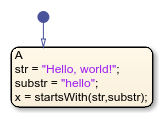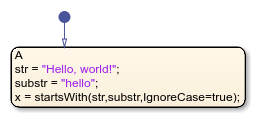# startsWith

## Syntax

``TF = startsWith(str,pat)``
``TF = startsWith(str,pat,'IgnoreCase',true)``

## Description

example

````TF = startsWith(str,pat)` returns `1` (`true`) if `str` starts with the specified pattern, and returns `0` (`false`) otherwise.```

example

````TF = startsWith(str,pat,'IgnoreCase',true)` ignores case when determining if `str` starts with `pat`. ```

Note

Only Stateflow® charts that use MATLAB® as the action language support the `startsWith` operator.

## Examples

expand all

```h = "Hello, world!" pat1 = "H" pat2 = "h" x = startsWith(h, pat1) x2 = startsWith(h, pat2) ``````h = "Hello, world!" pat1 = "H" pat2 = "h" x = startsWith(h, pat1, 'IgnoreCase', true) x2 = startsWith(h, pat2, 'IgnoreCase', true) ```## Limitations

### Topics

Introduced in R2021b

## SupportGet trial now